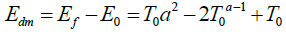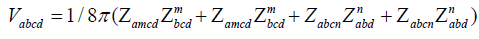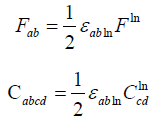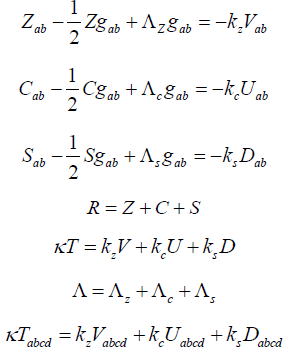Parası kalmadığı için otobüse binemiyordur ailesi porno izle ona daha yeni para gönderdiği için tekrar porno istemeye utanınca mecburen otostop çekmek için youporn çantasını alarak yol kenarına gelir etekli porno liseli türk kız yol kenarında dururken yanına yaklaşan porno kibar bir gencin onu gideceği yere kadar bırakmak porno izle istemesine çok mutlu olur arabaya bindiklerinde gideceği yer ile porno arabayı kullanan adamın gittiği yer arasında çok mesafe sex izle farkı olduğunu anlayan türk kız bu yaptığı porno indir iyilik karşısında arabada ona memelerini açar porno sapıklaşan adam yol kenarındaki hotelde durarak porno izle üniversiteli otostop çeken türk kızına odada sakso çektirip sikerOn Dark Matter Gravity Force Generated by the Expansion of the Universe
All submissions of the EM system will be redirected to Online Manuscript Submission System. Authors are requested to submit articles directly to Online Manuscript Submission System of respective journal.

Short communication
, Volume: 9( 3) DOI: 10.37532/23206756.2021.9(2).103

# On Dark Matter Gravity Force Generated by the Expansion of the Universe

*Correspondence:
Jesus Delso
Lapuerta Bachelor's Degree in Physics by Zaragoza University, Spain.
E-Mail: [email protected]

Received: March 04, 2021; Accepted: March 25, 2021; Published: April 04, 2021

Citation: Lapuerta JS. On Dark Matter Gravity Force Generated by the Expansion of the Universe. J Phys Astron. 2021;9(1):205.

### Abstract

Dark matter gravity force is generated by the expansion of the universe, gravitational force is not strong enough and an extra centripetal force is needed due to this expansion, energy related to this new force is stored in the dark matter energy tensor D(4,0) related to the Riemann curvature. The cosmological redshift energy loss due to the expansion of the universe is stored in the dark matter energy tensor D(4,0). The Cosmic Microwave Back- ground energy loss has been stored in the dark matter energy tensor D(4,0). Dark matter gravity is generated by D(4,0) energy related directly to the S(4,0) tensor, however this gravity is attributed to exotic particles never detected, galaxies in our universe are rotating with such speed that the gravity generated by their observable matter could not possibly hold them together. Total Energy T(4,0) and energy tensors are defined to complete the General Relativity field equations. The Ricci decomposition is a way of breaking up the Riemann curvature tensor into three orthogonal tensors, Z(4,0), Weyl tensor C(4,0) and S(4,0).

### Keywords

Dark matter; Gravity; Cosmic; Energy; Universe

### Dark Matter Gravity Force is generated by the Expansion of the Universe

In an expanding universe a satellite is in a uniform circular orbit around a planet, satellite mass m << planet mass M, initial radius r0, initial constant angular velocity ω0, initial velocity v0 << c, c the speed of light, due to the expansion of the universe the final radius rf = ar0, a>1, final constant angular velocity ωf , initial gravitational force F0g equals centripetal force.In the expansion of the universe angles remain invariant, so ω0 = ωf and gravitational force Fg equals centripetal forceSince ω<ω0, gravitational force Fg is not strong enough and an extra centripetal force is needed, this extra force is attributed to exotic particles never detectedDark Matter energy Edm has been stored in the dark matter energy tensor D(4,0) defined below, differentiating we get the dark matter force Fdm.### Cosmological Redshift Energy Loss due to the Expansion of the Universe

In an expanding closed universe, regardless our universe is closed or open, the final wave length of the photon when the closed universe reaches its maximum radius is :Where h is the Planck constant and El is the energy loss, in a closed universe after reaching its maximum expansion the universe comes backwards and will reach rnow again restoring the loss of energy to the photon, so this El has been stored and was not definitely lost.

The cosmological redshift energy loss due to the expansion of the universe is stored in the dark matter energy tensor D(4,0) related directly to the S(4,0) tensor, however this gravity is attributed to exotic particles never detected.

The Cosmic Microwave Background energy loss has been stored in the dark matter energy tensor D(4,0), gravitational waves and other fields are subject to the same redshift phenomena.

#### Conformal Energy U Defined as a Combination of C and the Hodge Dual of C, Dark Matter Energy D defined as a Combination of S and the Hodge Dual of S, Similar Definitions for V/Z and T/R

The Ricci decomposition is a way of breaking up the Riemann curvature tensor into three orthogonal tensors, Z, Weyl tensor C and S, S tensor generates the dark matter gravityWhere Rabcd is the Riemann tensor, Rab is the Ricci tensor, R is the Ricci scalar (the scalar curvature)

The conformal energy tensor U can be defined as a combination of C and the Hodge dual of CThe new dark matter energy tensor D can be defined as a combination of S and the Hodge dual of SThe new energy tensor V can be defined as a combination of Z and the Hodge dual of ZThe new Total Energy tensor T can be defined as a combination of the Riemann tensor R and the Hodge dual of R### Hodge Dual Definitions

The Hodge dual definition for Electromagnetic tensor and Weyl tensorThe Hodge dual definition for dark matter S tensor, Z and R tensorsWeyl tensor C(4,0) is related to the new Conformal Energy tensor U(4,0). Dark matter tensor S(4,0) is related to the new dark matter energy tensor D(4,0). Z(4,0) tensor is related to the new energy tensor V(4,0). Riemann ten-sor R(4,0) is related to the new Total Energy tensor T(4,0).

### Complete General Relativity Field Equations

The complete field equations are described by a new T(4,0) tensor for Total Energy, the new conformal energy tensor U(4,0), the new energy tensor V(4,0) and the new dark matter energy tensor D(4,0)In the general theory of relativity the Einstein field equations relate the geometry of space-time to the distribution of matter .### References

Welcome Message

###### Citations : 252

Journal of Physics & Astronomy received 252 citations as per Google Scholar report

#### Indexed In

• Open J Gate
• China National Knowledge Infrastructure (CNKI)
• Cosmos IF
• Directory of Research Journal Indexing (DRJI)
• MIAR
• Secret Search Engine Labs
• Euro Pub